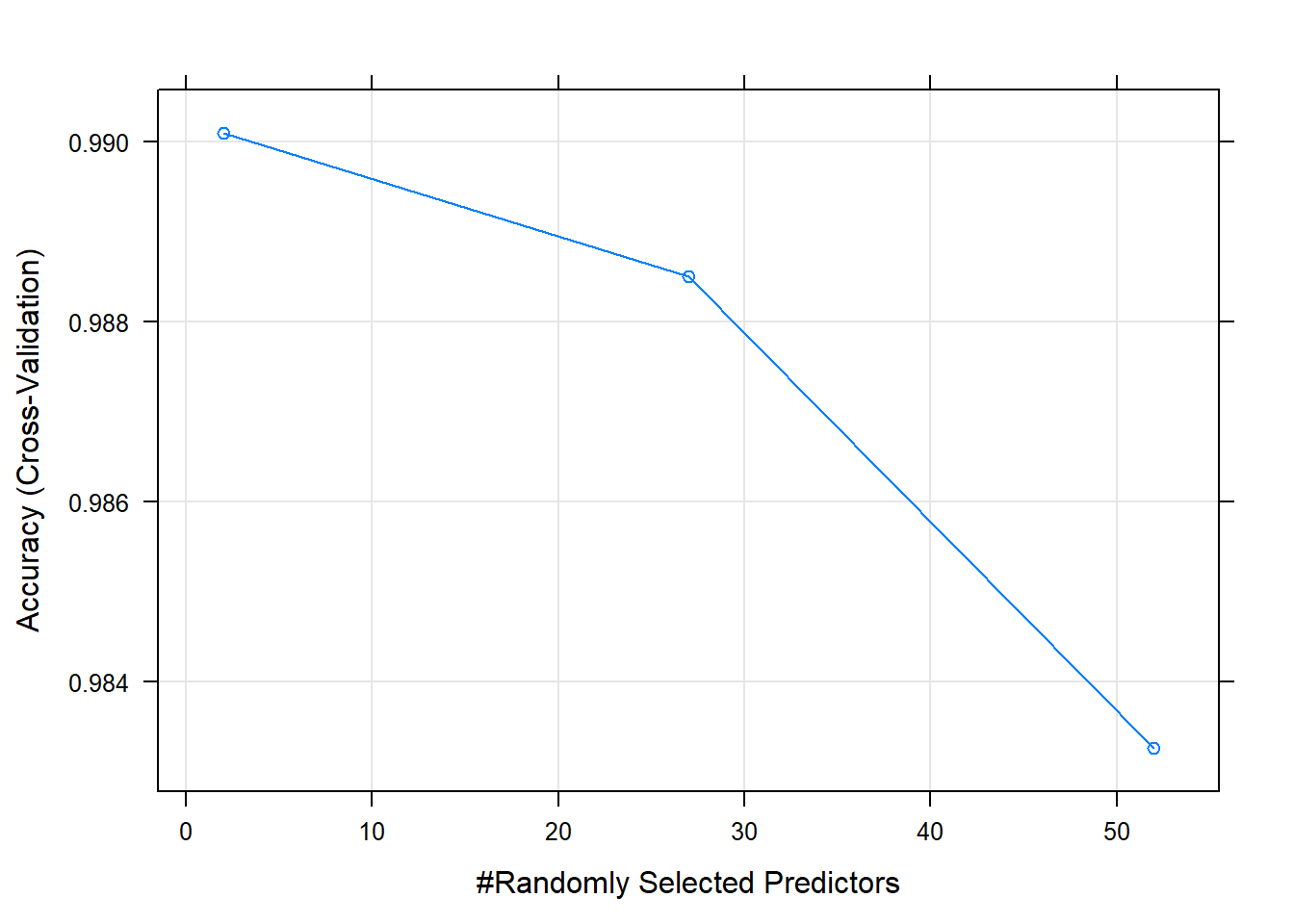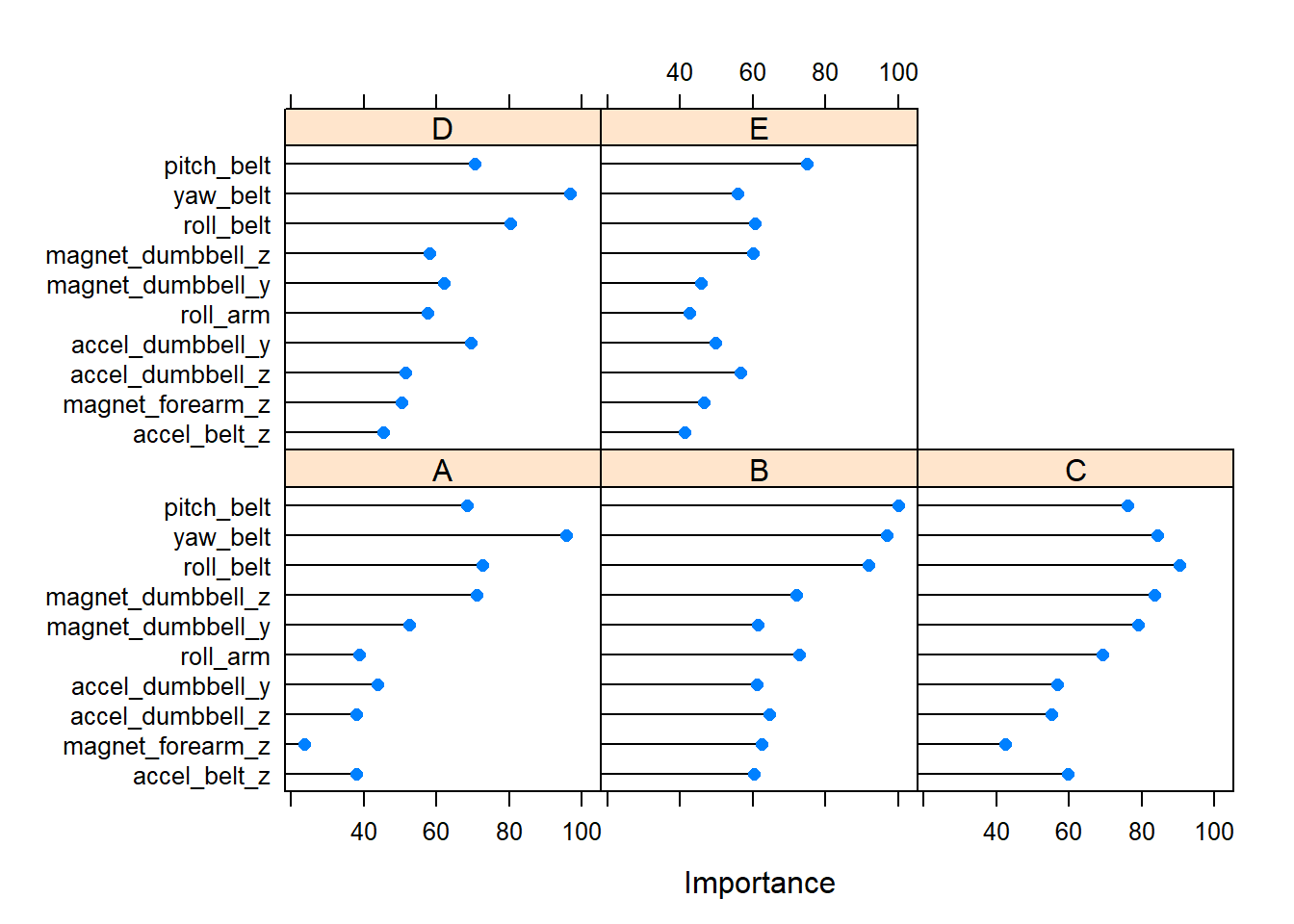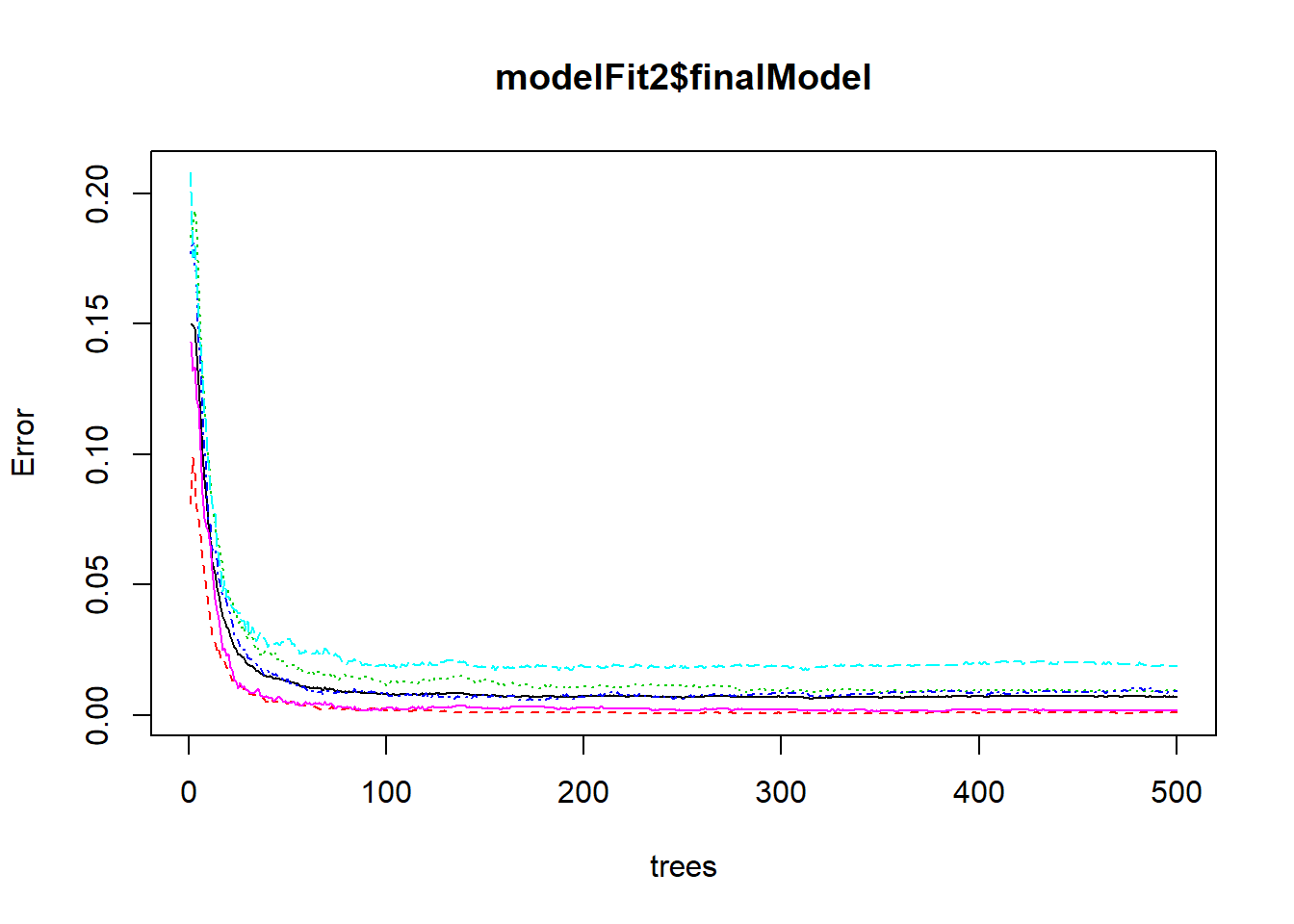## Summary

The goal of this exercise is to generate a prediction model based on the data collected by the human activity recognition research. This group provides a dataset with sports activity information collected on 8 hours of activities of six healthy subjects (information from the website). These six young health participants were asked to perform one set of 10 repetitions of the Unilateral Dumbbell Biceps Curl of 1.25kg in five different fashions: exactly according to the specification (Class A), throwing the elbows to the front (Class B), lifting the dumbbell only halfway (Class C), lowering the dumbbell only halfway (Class D) and throwing the hips to the front (Class E). Class A corresponds to the specified execution of the exercise, while the other 4 classes correspond to common mistakes(Velloso et al. 2013).

Our purpose here it to predict the manner in which they did the exercise based in some predictor variables.

There are some not-valid #DIV/0! values, we noticed the first time we read the dataset, so we replace them as NA values.

url1 <- "https://d396qusza40orc.cloudfront.net/predmachlearn/pml-training.csv"
trainingData = read.csv(url1,na.strings=c('#DIV/0', '', 'NA') ,stringsAsFactors = F)

url2 <- "https://d396qusza40orc.cloudfront.net/predmachlearn/pml-testing.csv"
testData = read.csv(url2,na.strings= c('#DIV/0', '', 'NA'),stringsAsFactors = F)

## Exploratory Analysis

We will perform first some exploratory analysis on the datasets. We need to perfom the same transformations in both the training and the test datasets. See outputs of the str command at the appendix of this document.

library(dplyr)
##
## Attaching package: 'dplyr'
## The following objects are masked from 'package:stats':
##
##     filter, lag
## The following objects are masked from 'package:base':
##
##     intersect, setdiff, setequal, union
str(trainingData)
str(testData)

There are NA values we need to clean. Let’s first decide what to do with these NA values.

sum(!complete.cases(trainingData))
##  19216

The number of incompleted cases is too high 19216, from the total 19622. Thus we cannot perfom a radical filter to eliminate all rows containing them because we would reduce too much the samples count. However we can delete the columns with such a huge number of NAs since they will be no good predictors anyway.

trainingCount <-sapply(trainingData, function(y) sum(length(which(is.na(y)))))
trainingCount$name <- rownames(trainingCount)  ## Warning in trainingCount$name <- rownames(trainingCount): Coercing LHS to a
## list
trainingCount <- data.frame(trainingCount)
# take columns with more than 19000 NA values
columns2Delete <- trainingCount[,apply(trainingCount, MARGIN =2, function(col){any(col>19000)})]
dim(columns2Delete)
##    1 100
# we have 100 columns to delete in the training set
reducedTrainingData <- trainingData[, ! names(trainingData) %in% names(columns2Delete)] 

We repeat the same transformation in the test set:

testCount <- sapply(testData, function(y) sum(length(which(is.na(y)))))
testCount$name <- rownames(testCount)  ## Warning in testCount$name <- rownames(testCount): Coercing LHS to a list
testCount <- data.frame(testCount)
# take columns with more than 19 NA values (the total number of rows is 20)
testCols2Delete <- testCount[,apply(testCount, MARGIN=2, function(col){any(col > 19)})]
reducedTestData <- testData[, ! names(testData) %in% names(testCols2Delete)] 

By checking the classes of the dataset we still have some columns as characters.

characterClasses <- names(reducedTrainingData[,sapply(reducedTrainingData,is.character)])

We have four columns user_name, cvtd_timestamp, new_window and classe. The second variable is a date so we transform it into the right type.

reducedTrainingData$cvtd_timestamp <- as.Date(reducedTrainingData$cvtd_timestamp, "%m/%d/%Y %H:%M")
reducedTestData$cvtd_timestamp <- as.Date(reducedTestData$cvtd_timestamp, "%m/%d/%Y %H:%M")

The classe variable is the outcome so we transform the other two into factors.

# transforming character variables into factors
reducedTrainingData$user_name <- as.factor(reducedTrainingData$user_name)
reducedTestData$user_name <- as.factor(reducedTestData$user_name)
reducedTrainingData$new_window <- as.factor(reducedTrainingData$new_window)
reducedTestData$new_window <- as.factor(reducedTestData$new_window)
sum(reducedTrainingData$new_window == "no") ##  19216 We highlighted that last variable, because from 19622 observations in the training set, the value no is in 19216 ones. Thus it is not going to bring a lot of information, but we will remove not-useful predictor in the next chapter. ## Prediction analysis ### Removing not useful predictors and preparing the datasets for the model All timestamp values are based on the time when the measure was taken, thus we consider them not relevant predictors, since we want to predict by analyzing the results of the performed activities. As we said before, the new_window does not help much in our predicting model, so we remove it too. The num_window is also removed since again is not related with the activities’ results. Besides the X column is just the row number so we also delete it. Finally at the test dataset there is a redundant problem_id column which is also the same as X , then we remove it too. In addition we decide to remove the user_name , since we want to predict just based on the results of the activity. # removing not useful predictors library(tidyverse) ## Loading tidyverse: ggplot2 ## Loading tidyverse: tibble ## Loading tidyverse: tidyr ## Loading tidyverse: readr ## Loading tidyverse: purrr ## Conflicts with tidy packages ---------------------------------------------- ## filter(): dplyr, stats ## lag(): dplyr, stats finalTrainingData <- reducedTrainingData %>% select(-X, -user_name, -cvtd_timestamp, -raw_timestamp_part_1, -raw_timestamp_part_2, -num_window, -new_window) finalTestData <- reducedTestData %>% select(-X, -user_name, -cvtd_timestamp, -problem_id, -raw_timestamp_part_1, -raw_timestamp_part_2, -num_window, -new_window) # put the outcome as factor finalTrainingData$classe <- as.factor(finalTrainingData$classe) We will keep the finalTestData for the final step of the assignment project. Notice that the test dataset has no classe column since it is our goal to predict it. Let’s divide the training set into two sets, the testing one will be used to check how good is our model. library(caret) ## Loading required package: lattice ## ## Attaching package: 'caret' ## The following object is masked from 'package:purrr': ## ## lift set.seed(1223) # create training and test sets 70% for training inTrain <- createDataPartition(y = finalTrainingData$classe,p = 0.7, list = FALSE)
training <- finalTrainingData[inTrain,]
testing <- finalTrainingData[-inTrain,]
# dimensions of the training set
dim(training)
##  13737    53

At the end we have 52 predictors, since one is the output variable. Let’s check briefly our predictors to check if we have Zero covariates. These are variables that have no variability at all and are not useful to construct a prediction model.

nearZeroVar(training,saveMetrics = TRUE)

We have no zero covariates so we keep all the predictors.

### Choosing a method

We choose random forest as is one of the most used/accurate algorithms along with boosting. We will use a Confusion Matrix to evaluate the accuracy of the model. When we tried to train the model with the default values, it did not end the computation. Thus we will improve the performance of the calculations using a parallel approach (as recommended here.

#### Configure parallel processing

library(parallel)
library(doParallel)
## Loading required package: foreach
##
## Attaching package: 'foreach'
## The following objects are masked from 'package:purrr':
##
##     accumulate, when
## Loading required package: iterators
cluster <- makeCluster(detectCores() - 1) # convention to leave 1 core for OS
registerDoParallel(cluster)

#### Cross validation

We will perform cross validation using the option trainControl of the train function at the caret package. We specify 3-fold cross-validation (10-fold is very time consuming). We use allowParallel = TRUE to accelerate the computing, which tells caret to use the registered cluster. We also add the importance flag to analyze the predictors.

stime <- system.time({
modelFit2 <- train(classe ~ ., data = training ,method ="rf", importance = T, trControl = trainControl(method="cv", number=3, verboseIter=FALSE), allowParallel=TRUE)
})
stime
## elapsed
##  552.06

The accuracy results are:

modelFit2
## Random Forest
##
## 13737 samples
##    52 predictor
##     5 classes: 'A', 'B', 'C', 'D', 'E'
##
## No pre-processing
## Resampling: Cross-Validated (3 fold)
## Summary of sample sizes: 9158, 9158, 9158
## Resampling results across tuning parameters:
##
##   mtry  Accuracy   Kappa
##    2    0.9900997  0.9874750
##   27    0.9884982  0.9854488
##   52    0.9832569  0.9788179
##
## Accuracy was used to select the optimal model using the largest value.
## The final value used for the model was mtry = 2.
plot(modelFit2)Thus it is clear from the graphic that more than 2 predictors does not improve the accuracy of the model.

#### De-register parallel processing cluster

stopCluster(cluster)
registerDoSEQ()

### Importance

We can identify the top 10 most important predictors.

vi <- varImp(modelFit2)
plot(vi, top = 10)### Sample error and accuracy

Now we predict the outcome for the test data set using the random forest model and check the accuracy again with the confusion matrix.

#### Confusion Matrix

library(caret)
pred <- predict(modelFit2, testing)
cmFit <- confusionMatrix(testing$classe, pred) cmFit ## Confusion Matrix and Statistics ## ## Reference ## Prediction A B C D E ## A 1674 0 0 0 0 ## B 5 1130 4 0 0 ## C 0 15 1010 1 0 ## D 0 0 16 946 2 ## E 0 0 0 1 1081 ## ## Overall Statistics ## ## Accuracy : 0.9925 ## 95% CI : (0.99, 0.9946) ## No Information Rate : 0.2853 ## P-Value [Acc > NIR] : < 2.2e-16 ## ## Kappa : 0.9905 ## Mcnemar's Test P-Value : NA ## ## Statistics by Class: ## ## Class: A Class: B Class: C Class: D Class: E ## Sensitivity 0.9970 0.9869 0.9806 0.9979 0.9982 ## Specificity 1.0000 0.9981 0.9967 0.9964 0.9998 ## Pos Pred Value 1.0000 0.9921 0.9844 0.9813 0.9991 ## Neg Pred Value 0.9988 0.9968 0.9959 0.9996 0.9996 ## Prevalence 0.2853 0.1946 0.1750 0.1611 0.1840 ## Detection Rate 0.2845 0.1920 0.1716 0.1607 0.1837 ## Detection Prevalence 0.2845 0.1935 0.1743 0.1638 0.1839 ## Balanced Accuracy 0.9985 0.9925 0.9886 0.9971 0.9990 ### Some plots By plotting the final model we see there was no improvement in the error with a number of trees higher than 100. The default value in the train function is 500. plot(modelFit2$finalModel)